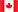## All Systems Operational

Since Tuesday, 23 February 2021, 04:00
Eastern Time Zone

• Careers
• Contact
•English (CA)Energy

# Calculating the right wattage for a room

29 June 2020

It would be useful to know the magic formula that would give us exactly the amount of heat required to heat a particular room or entire house. Fortunately, there are several formulas to get closer to the actual result but they come with a margin of error. Why the margin of error? It is due to the fact that not all houses are alike.

In order to calculate the heating required we must consider the size and volume of the house, orientation, size and number of windows, type of insulation found in the walls and roof, etc.

## TWO USEFUL FORMULAS

In general, the power required by electrical heating is calculated in watts.Wattage: Multiply the area in feet by 10. For a room 20 feet by 20 feet, we obtain 400 sq.ft., multiplied by 10 to give 4000 watts. The number of watts = area x 10.This result is valid for houses containing rooms whose ceilings are 8 feet high. In the case of modern homes having higher ceilings than 8 feet, the rule of thumb for the calculation is 1.25 watts per cubic foot. Taking into account the previous example, a 9-foot ceiling height would obtain 400 sq.ft x 9 x 1.25 = 4500 watts. The number of watts = area x height x 1.25.

If you suspect that the walls or ceilings have insulation deficiencies, feel free to add a few percentage points to the calculation. We can figure the same thing in the case of walls with oversized windows. After performing the calculations for an existing home, we may need to add extra heaters such as convectors or forced-air units.

Conversely, if a room is windowed and well oriented to the sun we can stay with the regular calculation.

The best assessment of the heating needs of a house will be done by the addition of the results for each room.

In North America we still can find BTU/hr being used as a measure of power in heating. The formula to convert BTU to kW is the following: P(kW)= P(BTU/hr)/ 3412.14.

If we rely solely on electric baseboards as a heating source, they are usually installed at the base of windows in order to get the best distribution of heat. In this case, do not hesitate to divide the total watts required by the number of the windows for each room.For more information on the type of heating equipment to use for a particular room or the complete house, please visit the following page.